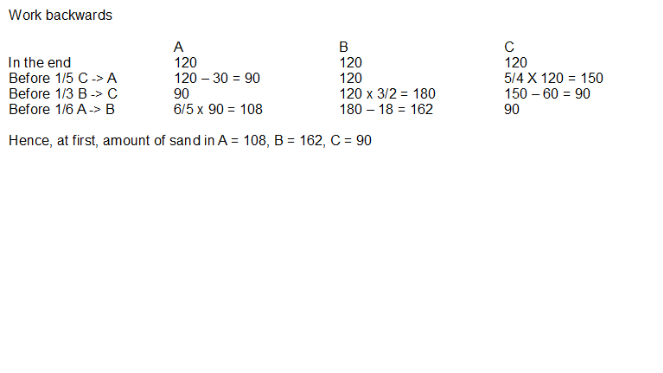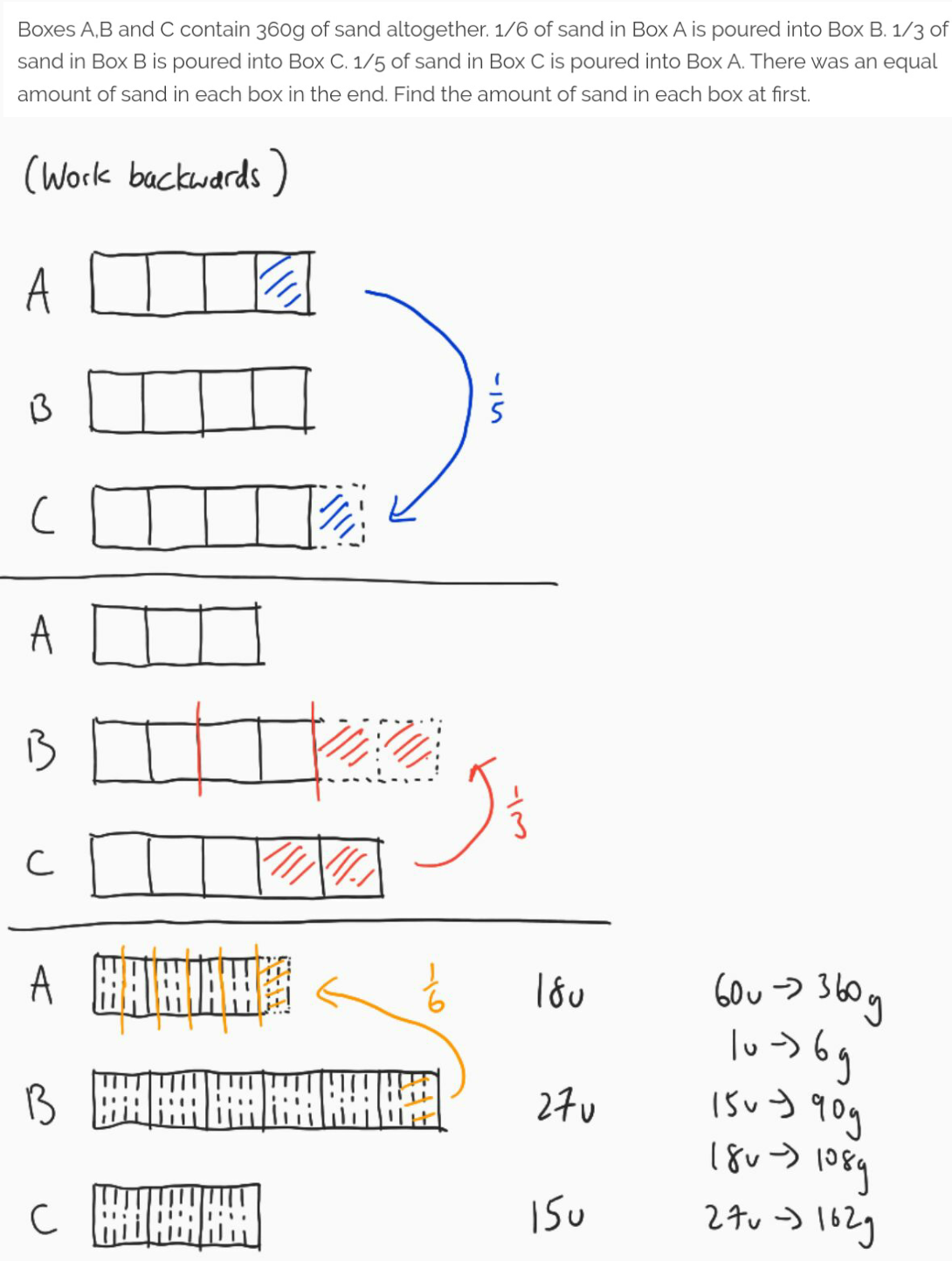# Question

Boxes A,B and C contain 360g of sand altogether. 1/6 of sand in Box A is poured into Box B. 1/3 of sand in Box B is poured into Box C. 1/5 of sand in Box C is poured into Box A. There was an equal amount of sand in each box in the end. Find the amount of sand in each box at first.

360/3 = 120

1/5 of sand in Box C is poured into Box A,
1 – 1/5 = 4/5
4/5 of sand in Box C ——- 120
1/5 of sand in Box C ——- 120/4 = 30
5/5 of sand in Box C ——- 30 x 5 = 150
Box C : 120 + 30 = 150
Box A : 120 – 30 = 90

1/3 of sand in Box B is poured into Box C,
1 – 1/3 = 2/3
2/3 of sand in Box B ——- 120
1/3 of sand in Box B ——- 120/2 = 60
3/3 of sand in Box B ——- 60 x 3 = 180
Box B : 120 + 60 = 180
Box C : 150 – 60 = 90

1/6 of sand in Box A is poured into Box B,
1 – 1/6 = 5/6
5/6 ——- 90
1/6 ——- 90/5 = 18
6/6 ——- 18 x 6 = 108
Box A : 90 + 18 = 108
Box B : 180 – 18 = 162

Ans : Box A : 108g, Box B : 162g and Box C : 90g.

0 Replies 1 Like0 Replies 1 Like0 Replies 1 Like